Courses

# Irodov Solutions: ELastic Deformations of A Solid Body- 2 Notes | EduRev

## JEE : Irodov Solutions: ELastic Deformations of A Solid Body- 2 Notes | EduRev

The document Irodov Solutions: ELastic Deformations of A Solid Body- 2 Notes | EduRev is a part of the JEE Course Physics For JEE.
All you need of JEE at this link: JEE

Q. 302.  A steel girder of length l rests freely on two supports (Fig. 1.77). The moment of inertia of its cross-section is equal to I (see the foregoing problem). Neglecting the mass of the girder and assuming the sagging to he slight, find the deflection λ due to the force F applied to the middle of the girder.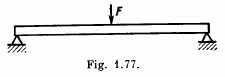Solution. 302. One can think of it as analogous to the previous case but with a beam of length l/2 loaded upward by a force F/2.

Thus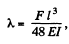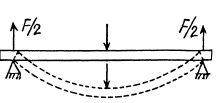On using the last result of the previous problem.

Q. 303. The thickness of a rectangular steel girder equals h. Using the equation of Problem 1.301, find the deflection λ caused by the weight of the girder in two cases:
(a) one end of the girder is embedded into a wall with the length of the protruding section being equal to l (Fig. 1.78a);
(b) the girder of length 2l rests freely on two supports (Fig. 1.78b).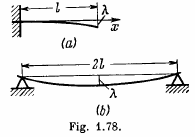Solution. 303.  (a)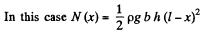where b = width of the girder.

Also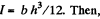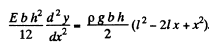Integrating,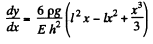using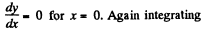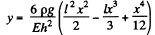Thus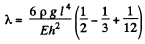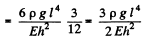(b) As before,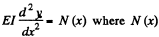is the bending moment due to section PB.

This bending moment is clearly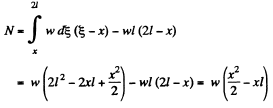(Here w = p g b h is weight o f the beam per unit length)

Now integrating,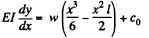or since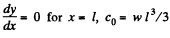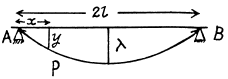Integrating again,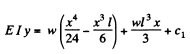As y = 0 for x = 0, c1 = 0. From this we find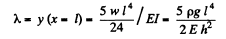Q. 304. A steel plate of thickness h has the shape of a square whose side equals l, with h <<  1. The plate is rigidly fixed to a vertical axle OO which is rotated with a constant angular acceleration 13 (Fig. 1.79). Find the deflection λ, assuming the sagging to be small.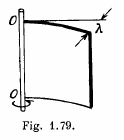Solution. 304. The deflection of the plate can be noticed by going to a co- rotating frame. In this frame each element of the plate experiences a pseudo force proportional to its mass. These forces have a moment which constitutes the bending moment of the problem. To calculate this moment we note that the acceleration of an element at a distance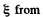from the axis is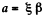and the moment of the forces exerted by the section between x and l is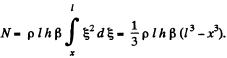From the fundamental equation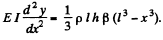The moment of inertia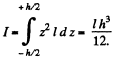Vofe that the neutral surface (i.e. the surface which contains lines which are neither stretched nor compressed) is a vertical plane here and z is perpendicular to it.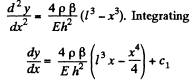Since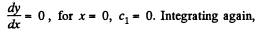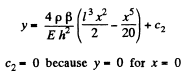Thus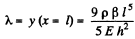Q. 305. Determine the relationship between the torque N and the torsion angle φ for
(a) the tube whose wall thickness Δr is considerably less than the tube radius;
(b) for the solid rod of circular cross-section. Their length l, radius r, and shear modulus G are supposed to be known.

Solution. 305. (a) Consider a hollow cylinder of length l, outer radius r + Δr inner radius r, fixed at one end and twisted at the other by means of a couple of moment N. The angular displacement φ, at a distance l from the fixed end, is proportional to both l and N. Consider an element of length dx at the twisted end. It is moved by an angle φ as shown. A vertical section is also shown and the twisting of the parallelopipe of length l and area Δr dx under the action of the twisting couple can be discussed by elementary means. If f is the tangential force generated then shearing stress is f/Δr dx and this must equal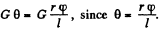Hence ,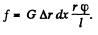The force / has moment f r about the axis and so the total moment is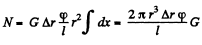(b) For a solid cylinder we must integrate over r. Thus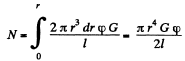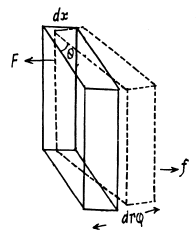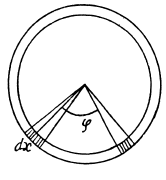Q. 306. Calculate the torque N twisting a steel tube of length l = 3.0 m through an angle φ = 2.0° about its axis, if the inside and outside diameters of the tube are equal to d1 =  30 mm and d2  = 50 mm.

Solution. 306.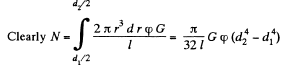using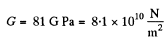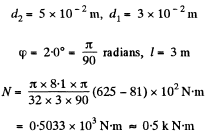Q. 307. Find the maximum power which can be transmitted by means of a steel shaft rotating about its axis with an angular velocity ω = 120 rad/s, if its length l = 200 cm, radius r = 1.50 cm, and the permissible torsion angle φ = 2.5°.

Solution. 307. The maximum power that can be transmitted by means of a shaft rotating about its axis is clearly N ω where N is the moment of the couple producing the maximum permissible torsion, φ. Thus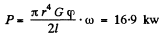Q. 308. A uniform ring of mass m, with the outside radius r2, is fitted tightly on a shaft of radius r1. The shaft is rotated about its axis with a constant angular acceleration β. Find the moment of elastic forces in the ring as a function of the distance r from the rotation axis.

Solution. 308. Consider an elementary ring of width dr at a distant r from the axis. The part outside exerts a couple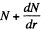dr on this ring while the part inside exerts a couple N in the opposite direction. We have for equilibrium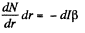where dl is the moment of inertia o f the elementary ring, β is the angular acceleration and minus sign is needed because the couple N (r) decreases, with distance vanshing at the outer radius, N (r2) = 0. Now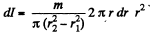Thus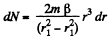or,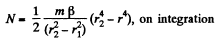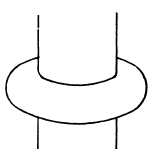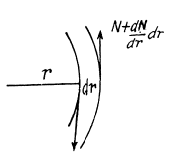Q. 309. Find the elastic deformation energy of a steel rod of mass m = 3.1 kg stretched to a tensile strain ε = 1.0-10-3

Solution. 309. We assume that the deformation is wholly due to external load, neglecting the effect of the weight of the rod (see next problem). Then a well known formula says,
elastic energy per unit volume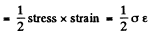This gives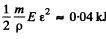for the total deformation energy.

Q. 310. A steel cylindrical rod of length l and radius r is suspended by its end from the ceiling.
(a) Find the elastic deformation energy U of the rod.
(b) Define U in terms of tensile strain Δl/l of the rod.

Solution. 310. When a rod is deformed by its own weight the stress increases as one moves up, the stretching force being the weight of the portion below the element considered.
The stress on the element dx is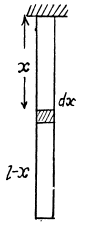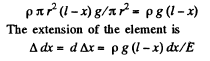Integrating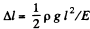is the extension of the whole rod. The elastic energy of the element is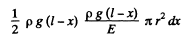Integrating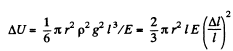Q. 311. What work has to be performed to make a hoop out of a steel band of length l = 2.0 m, width h = 6.0 cm, and thickness δ = 2.0 mm? The process is assumed to proceed within the elasticity range of the material.

Solution. 311. The work done to make a loop out of a steel band appears as the elastic energy of the loop and may be calculated from the same.

If the length of the band is l, the radius of the loop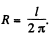Now consider an element ABCD of the loop. The elastic energy of this element can be calculated by the same sort of arguments as used to derive the formula for internal bending moment. Consider a fibre at a distance z from the neutral surface PQ. This fibre experiences a forcep and undergoes an extension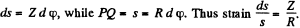If α is the cross sectional area of the fibre, the elastic energy associated with it is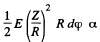Summing over all the fibres we get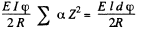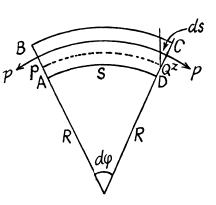For the whole loop this gives,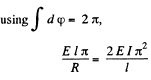Now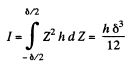So the energy is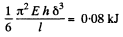Q. 312. Find the elastic deformation energy of a steel rod whose one end is fixed and the other is twisted through an angle φ = 6.0°. The length of the rod is equal to l = 1.0 m, and the radius to r = 10 mm.

Solution. 312. When the rod is twisted through an angle θ a couple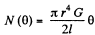appears to resist this. Work done in twisting the rod by an angle φ is then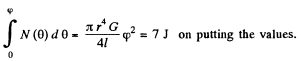Q. 313. Find how the volume density of the elastic deformation energy is distributed in a steel rod depending on the distance r from its axis. The length of the rod is equal to l, the torsion angle to φ.

Solution. 313. The energy between radii r and r + dr is, by differentiation,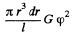Its density is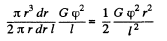Q. 314. Find the volume density of the elastic deformation energy in fresh water at the depth of h = 1000 m.

Solution. 314. The energy density is as usual 1/2 stress x strain. Stress is the pressure p g h. Strain is β x p gh by defination of β. Thus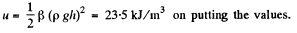Offer running on EduRev: Apply code STAYHOME200 to get INR 200 off on our premium plan EduRev Infinity!

## Physics For JEE

187 videos|516 docs|263 tests

,

,

,

,

,

,

,

,

,

,

,

,

,

,

,

,

,

,

,

,

,

;